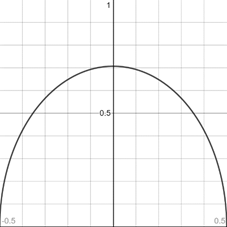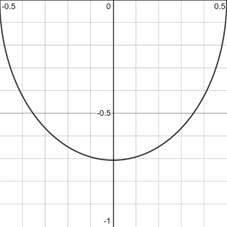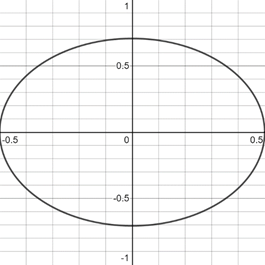# To graph : The ellipse 4 x 2 + 2 y 2 = 1 by solve for y and graphing two equations corresponding to the negative and positive square roots.### Precalculus: Mathematics for Calcu...

6th Edition
Stewart + 5 others
Publisher: Cengage Learning
ISBN: 9780840068071### Precalculus: Mathematics for Calcu...

6th Edition
Stewart + 5 others
Publisher: Cengage Learning
ISBN: 9780840068071

#### Solutions

Chapter 1.9, Problem 29E
To determine

## To graph: The ellipse 4x2+2y2=1 by solve for y and graphing two equations corresponding to the negative and positive square roots.

Expert Solution

### Explanation of Solution

Given information:

The equation of ellipse 4x2+2y2=1 .

Graph:

The graph of ellipse 4x2+2y2=1 by solving for y .

Subtract 4x2 from the equation of ellipse,

4x24x2+2y2=14x22y2=14x2

Divided by 2 in the equation,

y2=14x22

Take under root on both side of the equation,

y=±14x22

From the two equations graph of ellipse is described:

y=+14x22,y=14x22

The graph of the equation y=14x22 represents upper half of the ellipse because y is always greater than or equal to 0.

14x22014x2014x2±12x

So, the values of x is less than or equal to ±12 .

Thus, the graph appropriate in the [12,12]×[0,1] viewing rectangle

The graph of the equation y=14x22 can be sketched using the table,

 x y 0 12 18 1532 14 38

The graph of equation is provided below,The graph of the equation y=14x22 represents lower half of the ellipse because y is always less than or equal to 0.

14x22014x22014x20±12x

So, the values of x is greater than or equal to ±12 .

Thus, the graph appropriate in the [12,12]×[-1,0] viewing rectangle

The graph of the equation y=14x22 can be sketched using the table,

 x y 0 −12 12 0 14 −38

The graph of equation is provided below,the graph of ellipse 4x2+2y2=1 appropriate in the [12,12]×[-1,1] viewing rectangle

The graph of both equations in same figure is provided below,Interpretation:

The graph of an equation in a viewing screen is a viewing rectangle.

The x -values to range from a minimum value of xmin =a to a maximum value of xmax =b

The y -values to range from minimum value of ymin =c to a maximum value of ymax =d

Then, the display portion of the graph lies in the rectangle [a,b]×[c,d]={(x,y)|axb,cyd}

The graph of y=14x22 appropriate in the [12,12]×[0,1] viewing rectangle and graph make upper half ellipse.

The graph of y=14x22 appropriate in the [12,12]×[-1,0] viewing rectangle and graph make lower half ellipse.

### Have a homework question?

Subscribe to bartleby learn! Ask subject matter experts 30 homework questions each month. Plus, you’ll have access to millions of step-by-step textbook answers!